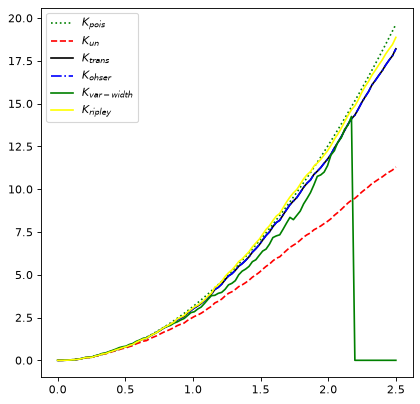# Ripley’s K Function Estimators¶

Spatial correlation functions have been used in the astronomical context to estimate the probability of finding an object (e.g., a galaxy) within a given distance of another object 1.

Ripley’s K function is a type of estimator used to characterize the correlation of such spatial point processes 2, 3, 4, 5, 6. More precisely, it describes correlation among objects in a given field. The `RipleysKEstimator` class implements some estimators for this function which provides several methods for edge effects correction.

## Basic Usage¶

The actual implementation of Ripley’s K function estimators lie in the method `evaluate`, which take the following arguments: `data`, `radii`, and optionally, `mode`.

The `data` argument is a 2D array which represents the set of observed points (events) in the area of study. The `radii` argument corresponds to a set of distances for which the estimator will be evaluated. The `mode` argument takes a value on the following linguistic set `{none, translation, ohser, var-width, ripley}`; each keyword represents a different method to perform correction due to edge effects. See the API documentation and references for details about these methods.

Instances of `RipleysKEstimator` can also be used as callables (which is equivalent to calling the `evaluate` method).

### Example¶

To use Ripley’s K Function Estimators from `astropy`’s stats sub-package:

```import numpy as np
from matplotlib import pyplot as plt
from astropy.stats import RipleysKEstimator

rng = np.random.default_rng()
z = rng.uniform(low=5, high=10, size=(100, 2))
Kest = RipleysKEstimator(area=25, x_max=10, y_max=10, x_min=5, y_min=5)

r = np.linspace(0, 2.5, 100)
plt.plot(r, Kest.poisson(r), color='green', ls=':', label=r'\$K_{pois}\$')
plt.plot(r, Kest(data=z, radii=r, mode='none'), color='red', ls='--',
label=r'\$K_{un}\$')
plt.plot(r, Kest(data=z, radii=r, mode='translation'), color='black',
label=r'\$K_{trans}\$')
plt.plot(r, Kest(data=z, radii=r, mode='ohser'), color='blue', ls='-.',
label=r'\$K_{ohser}\$')
plt.plot(r, Kest(data=z, radii=r, mode='var-width'), color='green',
label=r'\$K_{var-width}\$')
plt.plot(r, Kest(data=z, radii=r, mode='ripley'), color='yellow',
label=r'\$K_{ripley}\$')
plt.legend()
```

(png, svg, pdf)